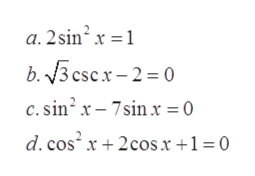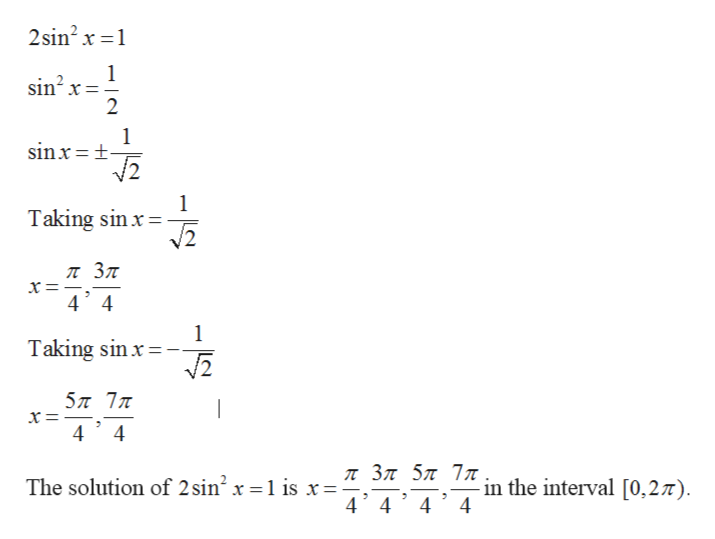# In each of the equation below, find all solutions [0, 2π)a. 2sin2x=1b. √3 cscxm - 2=0c. sin2x-7sinx=0d. cos2x+2cosx+1=0

Question
4 views

In each of the equation below, find all solutions [0, 2π)

a. 2sin2x=1

b. √3 cscxm - 2=0

c. sin2x-7sinx=0

d. cos2x+2cosx+1=0

check_circle

Step 1

Given: -help_outlineImage Transcriptionclosea. 2sin? x = 1 b. 3 csc x - 2 = 0 c. sin? x – 7sin x = 0 d. cos x + 2cos x +1=0 fullscreen
Step 2

To find: -

Step 3

Calculation: -

Pa...help_outlineImage Transcriptionclose2sin? x =1 sin? x=: sin x=±- V2 Taking sin x = л Зл x=-,- 4 4 Taking sin x = 5л 7л x= - л Зл 5л 7л 4' 4'4' 4 The solution of 2 sin x =1 is x= in the interval [0,27). fullscreen

### Want to see the full answer?

See Solution

#### Want to see this answer and more?

Solutions are written by subject experts who are available 24/7. Questions are typically answered within 1 hour.*

See Solution
*Response times may vary by subject and question.
Tagged in

### Calculus